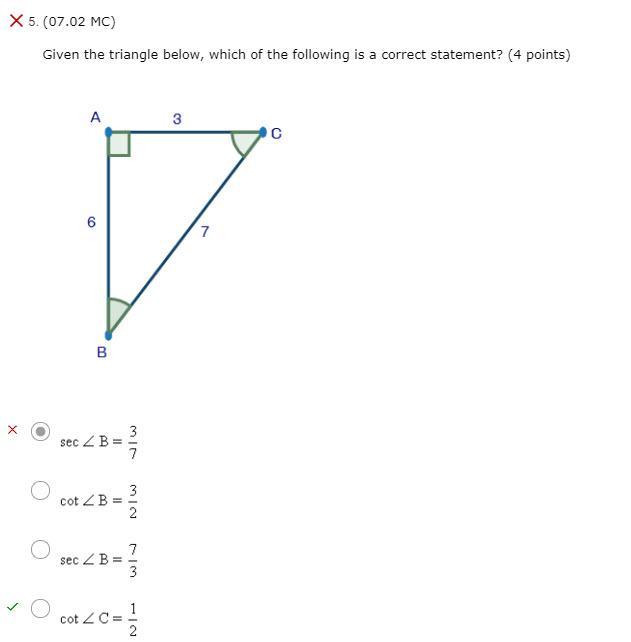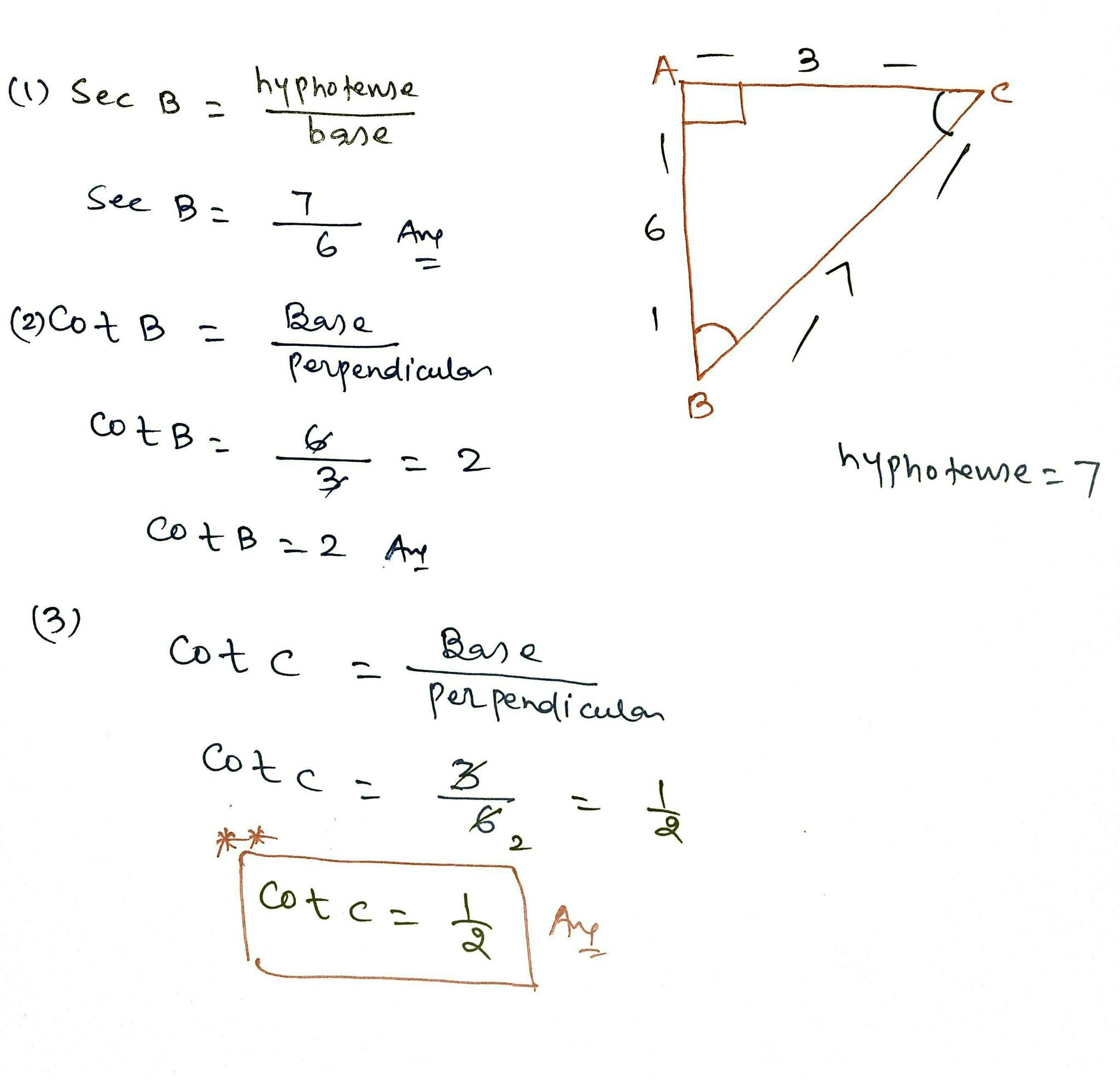# Given the triangle below. which of the following is a correct statement?X 5. (07.02 MC) Given the triangle below, which of the following is a correct statement? (4 points) A 3 3 С 6 7 B X sec ZB 3 7 3 cot B = 2 sec ZB 7 3 cot ZCE 1 2

1 A 3 (1) Sec B = hyphofense base s See B= 7 6 Ane 6 7 (2) Cot B I Base perpendiculan cotB= 3 6 – 2 hyphotense=7 30 cotB = 2 Ang (3) Cotc Base perpendiculan Cotca 3 6 2 cotca let